# 5.3 Graphs of polynomial functions  (Page 9/13)

 Page 9 / 13

$f\left(x\right)={x}^{5}-2x,\text{\hspace{0.17em}}$ between $\text{\hspace{0.17em}}x=1\text{\hspace{0.17em}}$ and $\text{\hspace{0.17em}}x=2.$

$f\left(x\right)=-{x}^{4}+4,\text{\hspace{0.17em}}$ between $\text{\hspace{0.17em}}x=1\text{\hspace{0.17em}}$ and $\text{\hspace{0.17em}}x=3$ .

$f\left(1\right)=3\text{\hspace{0.17em}}$ and $\text{\hspace{0.17em}}f\left(3\right)=–77.\text{\hspace{0.17em}}$ Sign change confirms.

$f\left(x\right)=-2{x}^{3}-x,\text{\hspace{0.17em}}$ between $\text{\hspace{0.17em}}x=–1\text{\hspace{0.17em}}$ and $\text{\hspace{0.17em}}x=1.$

$f\left(x\right)={x}^{3}-100x+2,\text{\hspace{0.17em}}$ between $\text{\hspace{0.17em}}x=0.01\text{\hspace{0.17em}}$ and $\text{\hspace{0.17em}}x=0.1$

$f\left(0.01\right)=1.000001\text{\hspace{0.17em}}$ and $\text{\hspace{0.17em}}f\left(0.1\right)=–7.999.\text{\hspace{0.17em}}$ Sign change confirms.

For the following exercises, find the zeros and give the multiplicity of each.

$f\left(x\right)={\left(x+2\right)}^{3}{\left(x-3\right)}^{2}$

$f\left(x\right)={x}^{2}{\left(2x+3\right)}^{5}{\left(x-4\right)}^{2}$

0 with multiplicity 2, $\text{\hspace{0.17em}}-\frac{3}{2}\text{\hspace{0.17em}}$ with multiplicity 5, 4 with multiplicity 2

$f\left(x\right)={x}^{3}{\left(x-1\right)}^{3}\left(x+2\right)$

$f\left(x\right)={x}^{2}\left({x}^{2}+4x+4\right)$

0 with multiplicity 2, –2 with multiplicity 2

$f\left(x\right)={\left(2x+1\right)}^{3}\left(9{x}^{2}-6x+1\right)$

$f\left(x\right)={\left(3x+2\right)}^{5}\left({x}^{2}-10x+25\right)$

$-\frac{2}{3}\text{\hspace{0.17em}}\text{with}\text{\hspace{0.17em}}\text{multiplicity}\text{\hspace{0.17em}}5\text{,}\text{\hspace{0.17em}}5\text{\hspace{0.17em}}\text{with}\text{\hspace{0.17em}}\text{multiplicity}\text{\hspace{0.17em}}\text{2}$

$f\left(x\right)=x\left(4{x}^{2}-12x+9\right)\left({x}^{2}+8x+16\right)$

$f\left(x\right)={x}^{6}-{x}^{5}-2{x}^{4}$

$\text{0}\text{\hspace{0.17em}}\text{with}\text{\hspace{0.17em}}\text{multiplicity}\text{\hspace{0.17em}}4\text{,}\text{\hspace{0.17em}}2\text{\hspace{0.17em}}\text{with}\text{\hspace{0.17em}}\text{multiplicity}\text{\hspace{0.17em}}1\text{,}\text{\hspace{0.17em}}–\text{1}\text{\hspace{0.17em}}\text{with}\text{\hspace{0.17em}}\text{multiplicity}\text{\hspace{0.17em}}1$

$f\left(x\right)=3{x}^{4}+6{x}^{3}+3{x}^{2}$

$f\left(x\right)=4{x}^{5}-12{x}^{4}+9{x}^{3}$

$\frac{3}{2}\text{\hspace{0.17em}}$ with multiplicity 2, 0 with multiplicity 3

$f\left(x\right)=2{x}^{4}\left({x}^{3}-4{x}^{2}+4x\right)$

$f\left(x\right)=4{x}^{4}\left(9{x}^{4}-12{x}^{3}+4{x}^{2}\right)$

$\text{0}\text{\hspace{0.17em}}\text{with}\text{\hspace{0.17em}}\text{multiplicity}\text{\hspace{0.17em}}6\text{,}\text{\hspace{0.17em}}\frac{2}{3}\text{\hspace{0.17em}}\text{with}\text{\hspace{0.17em}}\text{multiplicity}\text{\hspace{0.17em}}2$

## Graphical

For the following exercises, graph the polynomial functions. Note $\text{\hspace{0.17em}}x\text{-}$ and $\text{\hspace{0.17em}}y\text{-}$ intercepts, multiplicity, and end behavior.

$f\left(x\right)={\left(x+3\right)}^{2}\left(x-2\right)$

$g\left(x\right)=\left(x+4\right){\left(x-1\right)}^{2}$

x -intercepts, $\left(1, 0\right)$ with multiplicity 2, with multiplicity 1, $y\text{-}$ intercept As $\phantom{\rule{0.2em}{0ex}}x\to -\infty ,\phantom{\rule{0.2em}{0ex}}f\left(x\right)\to -\infty ,\text{\hspace{0.17em}}\text{as}\phantom{\rule{0.2em}{0ex}}x\to \infty ,\phantom{\rule{0.2em}{0ex}}f\left(x\right)\to \infty .$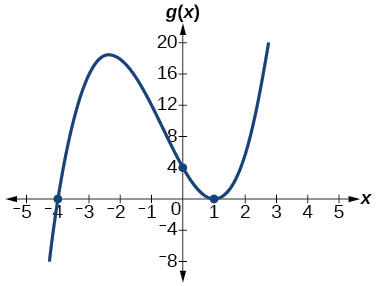$h\left(x\right)={\left(x-1\right)}^{3}{\left(x+3\right)}^{2}$

$k\left(x\right)={\left(x-3\right)}^{3}{\left(x-2\right)}^{2}$

x -intercepts $\text{\hspace{0.17em}}\left(3,0\right)\text{\hspace{0.17em}}$ with multiplicity 3, $\text{\hspace{0.17em}}\left(2,0\right)\text{\hspace{0.17em}}$ with multiplicity 2, $\text{\hspace{0.17em}}y\text{-}$ intercept $\text{\hspace{0.17em}}\left(0,–108\right).\text{\hspace{0.17em}}$ As $x\to -\infty ,\phantom{\rule{0.2em}{0ex}}f\left(x\right)\to -\infty ,\phantom{\rule{0.2em}{0ex}}\text{as}\phantom{\rule{0.2em}{0ex}}x\to \infty ,\phantom{\rule{0.2em}{0ex}}f\left(x\right)\to \infty .$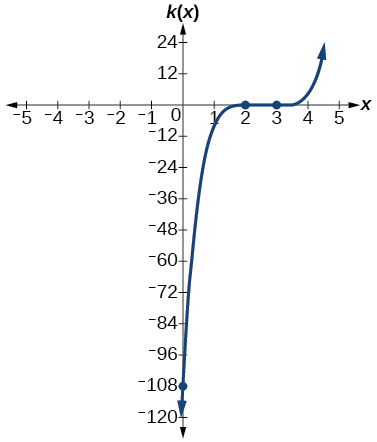$m\left(x\right)=-2x\left(x-1\right)\left(x+3\right)$

$n\left(x\right)=-3x\left(x+2\right)\left(x-4\right)$

x -intercepts with multiplicity 1, $\text{\hspace{0.17em}}y\text{-}$ intercept As $x\to -\infty ,\phantom{\rule{0.2em}{0ex}}f\left(x\right)\to \infty ,\phantom{\rule{0.2em}{0ex}}\text{as}\phantom{\rule{0.2em}{0ex}}x\to \infty ,\phantom{\rule{0.2em}{0ex}}f\left(x\right)\to -\infty .$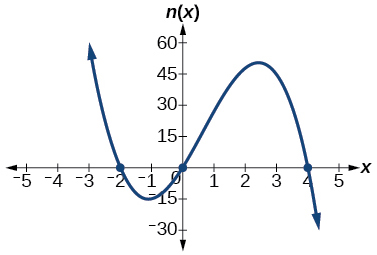For the following exercises, use the graphs to write the formula for a polynomial function of least degree.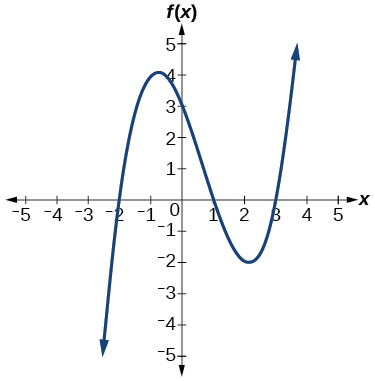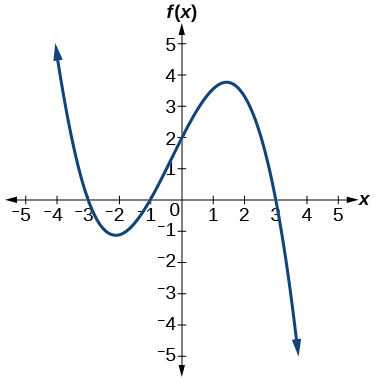$f\left(x\right)=-\frac{2}{9}\left(x-3\right)\left(x+1\right)\left(x+3\right)$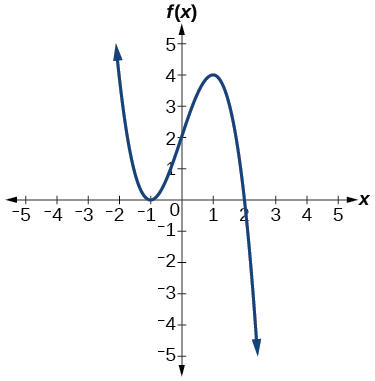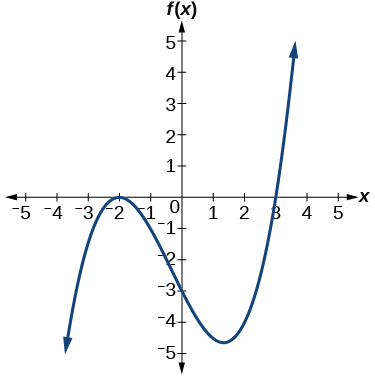$f\left(x\right)=\frac{1}{4}{\left(x+2\right)}^{2}\left(x-3\right)$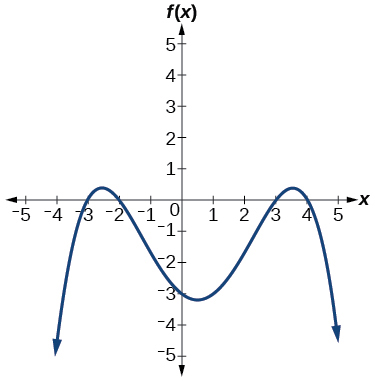For the following exercises, use the graph to identify zeros and multiplicity.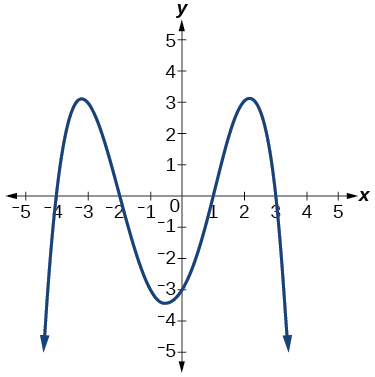–4, –2, 1, 3 with multiplicity 1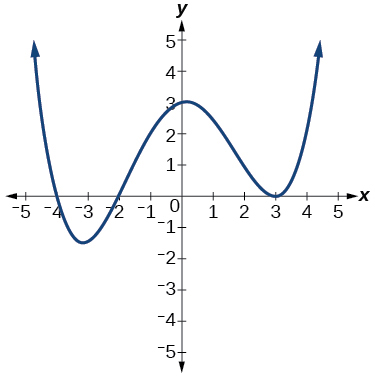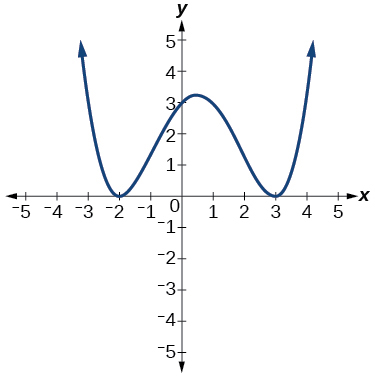–2, 3 each with multiplicity 2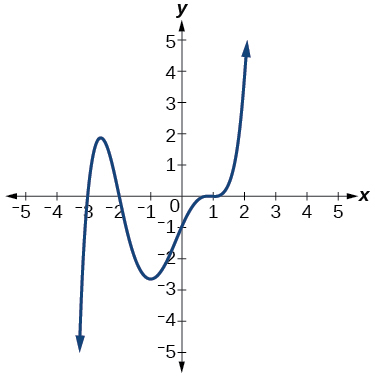For the following exercises, use the given information about the polynomial graph to write the equation.

Degree 3. Zeros at $\text{\hspace{0.17em}}x=–2,$ $\text{\hspace{0.17em}}x=1,\text{\hspace{0.17em}}$ and $\text{\hspace{0.17em}}x=3.\text{\hspace{0.17em}}$ y -intercept at $\text{\hspace{0.17em}}\left(0,–4\right).$

$f\left(x\right)=-\frac{2}{3}\left(x+2\right)\left(x-1\right)\left(x-3\right)$

Degree 3. Zeros at $\text{\hspace{0.17em}}x=\text{–5,}$ $\text{\hspace{0.17em}}x=–2,$ and $\text{\hspace{0.17em}}x=1.\text{\hspace{0.17em}}$ y -intercept at $\text{\hspace{0.17em}}\left(0,6\right)$

Degree 5. Roots of multiplicity 2 at $\text{\hspace{0.17em}}x=3\text{\hspace{0.17em}}$ and $\text{\hspace{0.17em}}x=1\text{\hspace{0.17em}}$ , and a root of multiplicity 1 at $\text{\hspace{0.17em}}x=–3.\text{\hspace{0.17em}}$ y -intercept at $\text{\hspace{0.17em}}\left(0,9\right)$

$f\left(x\right)=\frac{1}{3}{\left(x-3\right)}^{2}{\left(x-1\right)}^{2}\left(x+3\right)$

Degree 4. Root of multiplicity 2 at $\text{\hspace{0.17em}}x=4,\text{\hspace{0.17em}}$ and a roots of multiplicity 1 at $\text{\hspace{0.17em}}x=1\text{\hspace{0.17em}}$ and $\text{\hspace{0.17em}}x=–2.\text{\hspace{0.17em}}$ y -intercept at $\text{\hspace{0.17em}}\left(0,\text{–}3\right).$

Degree 5. Double zero at $\text{\hspace{0.17em}}x=1,\text{\hspace{0.17em}}$ and triple zero at $\text{\hspace{0.17em}}x=3.\text{\hspace{0.17em}}$ Passes through the point $\text{\hspace{0.17em}}\left(2,15\right).$

$f\left(x\right)=-15{\left(x-1\right)}^{2}{\left(x-3\right)}^{3}$

Degree 3. Zeros at $\text{\hspace{0.17em}}x=4,$ $\text{\hspace{0.17em}}x=3,$ and $\text{\hspace{0.17em}}x=2.\text{\hspace{0.17em}}$ y -intercept at $\text{\hspace{0.17em}}\left(0,-24\right).$

Degree 3. Zeros at $\text{\hspace{0.17em}}x=-3,$ $\text{\hspace{0.17em}}x=-2\text{\hspace{0.17em}}$ and $\text{\hspace{0.17em}}x=1.\text{\hspace{0.17em}}$ y -intercept at $\text{\hspace{0.17em}}\left(0,12\right).$

$f\left(x\right)=-2\left(x+3\right)\left(x+2\right)\left(x-1\right)$

Degree 5. Roots of multiplicity 2 at $\text{\hspace{0.17em}}x=-3\text{\hspace{0.17em}}$ and $\text{\hspace{0.17em}}x=2\text{\hspace{0.17em}}$ and a root of multiplicity 1 at $\text{\hspace{0.17em}}x=-2.$

y -intercept at

Degree 4. Roots of multiplicity 2 at $\text{\hspace{0.17em}}x=\frac{1}{2}\text{\hspace{0.17em}}$ and roots of multiplicity 1 at $\text{\hspace{0.17em}}x=6\text{\hspace{0.17em}}$ and $\text{\hspace{0.17em}}x=-2.$

y -intercept at $\text{\hspace{0.17em}}\left(0,18\right).$

$f\left(x\right)=-\frac{3}{2}{\left(2x-1\right)}^{2}\left(x-6\right)\left(x+2\right)$

Double zero at $\text{\hspace{0.17em}}x=-3\text{\hspace{0.17em}}$ and triple zero at $\text{\hspace{0.17em}}x=0.\text{\hspace{0.17em}}$ Passes through the point $\text{\hspace{0.17em}}\left(1,32\right).$

## Technology

For the following exercises, use a calculator to approximate local minima and maxima or the global minimum and maximum.

$f\left(x\right)={x}^{3}-x-1$

local max local min

$f\left(x\right)=2{x}^{3}-3x-1$

$f\left(x\right)={x}^{4}+x$

global min

$f\left(x\right)=-{x}^{4}+3x-2$

$f\left(x\right)={x}^{4}-{x}^{3}+1$

global min

## Extensions

For the following exercises, use the graphs to write a polynomial function of least degree.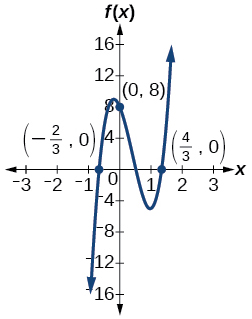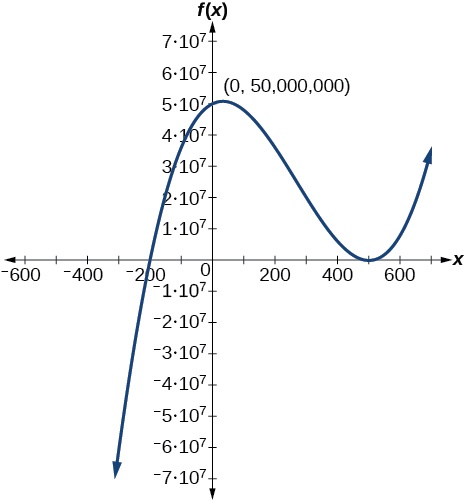$f\left(x\right)={\left(x-500\right)}^{2}\left(x+200\right)$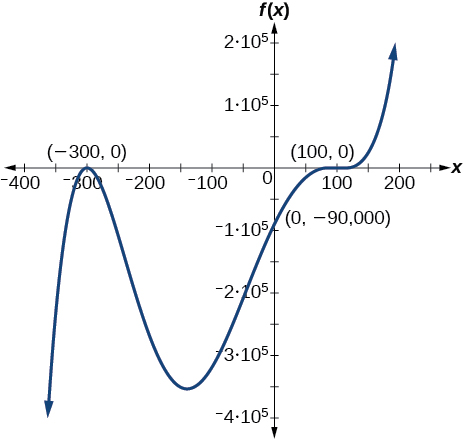## Real-world applications

For the following exercises, write the polynomial function that models the given situation.

A rectangle has a length of 10 units and a width of 8 units. Squares of $\text{\hspace{0.17em}}x\text{\hspace{0.17em}}$ by $\text{\hspace{0.17em}}x\text{\hspace{0.17em}}$ units are cut out of each corner, and then the sides are folded up to create an open box. Express the volume of the box as a polynomial function in terms of $\text{\hspace{0.17em}}x.$

$f\left(x\right)=4{x}^{3}-36{x}^{2}+80x$

Consider the same rectangle of the preceding problem. Squares of $\text{\hspace{0.17em}}2x\text{\hspace{0.17em}}$ by $\text{\hspace{0.17em}}2x\text{\hspace{0.17em}}$ units are cut out of each corner. Express the volume of the box as a polynomial in terms of $\text{\hspace{0.17em}}x.$

A square has sides of 12 units. Squares by units are cut out of each corner, and then the sides are folded up to create an open box. Express the volume of the box as a function in terms of $\text{\hspace{0.17em}}x.$

$f\left(x\right)=4{x}^{3}-36{x}^{2}+60x+100$

A cylinder has a radius of $\text{\hspace{0.17em}}x+2\text{\hspace{0.17em}}$ units and a height of 3 units greater. Express the volume of the cylinder as a polynomial function.

A right circular cone has a radius of $\text{\hspace{0.17em}}3x+6\text{\hspace{0.17em}}$ and a height 3 units less. Express the volume of the cone as a polynomial function. The volume of a cone is $\text{\hspace{0.17em}}V=\frac{1}{3}\pi {r}^{2}h\text{\hspace{0.17em}}$ for radius $\text{\hspace{0.17em}}r\text{\hspace{0.17em}}$ and height $\text{\hspace{0.17em}}h.$

$f\left(x\right)=\pi \left(9{x}^{3}+45{x}^{2}+72x+36\right)$

what is the function of sine with respect of cosine , graphically
tangent bruh
Steve
cosx.cos2x.cos4x.cos8x
sinx sin2x is linearly dependent
what is a reciprocal
The reciprocal of a number is 1 divided by a number. eg the reciprocal of 10 is 1/10 which is 0.1
Shemmy
Reciprocal is a pair of numbers that, when multiplied together, equal to 1. Example; the reciprocal of 3 is ⅓, because 3 multiplied by ⅓ is equal to 1
Jeza
each term in a sequence below is five times the previous term what is the eighth term in the sequence
I don't understand how radicals works pls
How look for the general solution of a trig function
stock therom F=(x2+y2) i-2xy J jaha x=a y=o y=b
sinx sin2x is linearly dependent
cr
root under 3-root under 2 by 5 y square
The sum of the first n terms of a certain series is 2^n-1, Show that , this series is Geometric and Find the formula of the n^th
cosA\1+sinA=secA-tanA
Wrong question
why two x + seven is equal to nineteen.
The numbers cannot be combined with the x
Othman
2x + 7 =19
humberto
2x +7=19. 2x=19 - 7 2x=12 x=6
Yvonne
because x is 6
SAIDI
what is the best practice that will address the issue on this topic? anyone who can help me. i'm working on my action research.
simplify each radical by removing as many factors as possible (a) √75
how is infinity bidder from undefined?ByByBy OpenStaxBy OpenStaxBy OpenStaxBy Janet ForresterBy Sebastian Sieczko...By OpenStaxBy OpenStaxBy Rohini AjayBy Lakeima RobertsBy Jonathan Long